## Example Questions

### Example Question #1 : How To Find The Reciprocal Of A Fraction

What is the reciprocal of the fraction 3/727?

727/3

3/727

–3/727

242

–727/3

727/3

Explanation:

The reciprocal of a fraction is just switching the numerator (top number) and the denominator (bottom number). The negative reciprocal takes the negative of that number.

3/727

Reciprocal 727/3

### Example Question #2 : How To Find The Reciprocal Of A Fraction

What is the opposite reciprocal of 25/127?

–127/25

–5

127/25

–25/127

25/127

–127/25

Explanation:

The reciprocal of a fraction is just switching the numerator (top number) and the denominator (bottom number).  The opposite reciprocal takes the negative of that number.

### Example Question #3 : How To Find The Reciprocal Of A Fraction

Find the negative reciprocal of the following: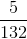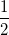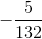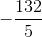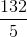Explanation:

The reciprocal of a fraction is just switching the numerator (top number) and the denominator (bottom number).  The negative reciprocal takes the negative of that number.ReciprocalNegative Reciprocal### Example Question #4 : How To Find The Reciprocal Of A Fraction

What is the slope of any line perpendicular to 4y = 2x + 7 ?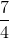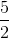Explanation:

First, we must solve the equation for y to determine the slope:  y = (2/4)x + 7/4

By looking at the coefficient in front of x, we know that the slope of this line has a value of 1/2. To find the slope of any line perpendicular to this one, we take the negative reciprocal of it:

slope = m , perpendicular slope = –1/m

slope = 1/2 , perpendicular slope = –2

### Example Question #5 : How To Find The Reciprocal Of A Fraction

Find the reciprocal of the following fraction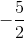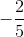Explanation:

The reciprocal is defined such that a faction times its recprocal is 1. For you this just means turn the fraction upside down, ie the numerator is the denominator and vice versa.

### Example Question #6 : How To Find The Reciprocal Of A Fraction

What is the reciprocal of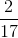?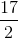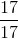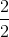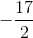This fraction doesn't have a reciprocal.Explanation:

The reciprocal of a fraction is simply exchanging the denominator and numerator of a fraction.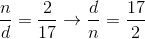You can double check that it works by making sure the product of a fraction and its reciprocal is 1.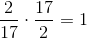### All ACT Math Resources